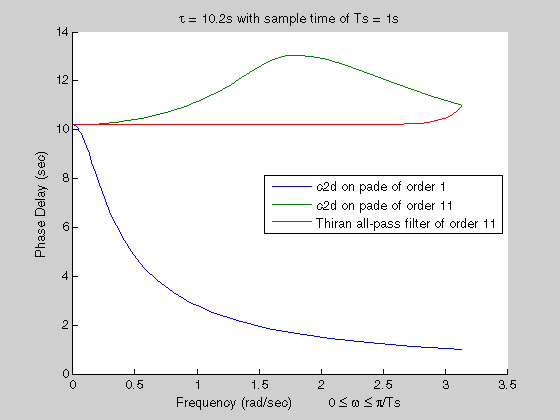## Time-Delay Approximation

Many control design algorithms cannot handle time delays directly. For example, techniques such as root locus, LQG, and pole placement do not work properly if time delays are present. A common technique is to replace delays with all-pass filters that approximate the delays.

To approximate time delays in continuous-time LTI models, use the `pade` command to compute a Padé approximation. The Padé approximation is valid only at low frequencies, and provides better frequency-domain approximation than time-domain approximation. It is therefore important to compare the true and approximate responses to choose the right approximation order and check the approximation validity.

### Time-Delay Approximation in Discrete-Time Models

For discrete-time models, use `absorbDelay` to convert a time delay to factors of 1/z where the time delay is an integer multiple of the sample time.

Use the `thiran` command to approximate a time delay that is a fractional multiple of the sample time as a Thiran all-pass filter.

For a time delay of `tau` and a sample time of `Ts`, the syntax `thiran(tau,Ts)` creates a discrete-time transfer function that is the product of two terms:

• A term representing the integer portion of the time delay as a pure line delay, (1/z)N, where `N = ceil(tau/Ts)`.

• A term approximating the fractional portion of the time delay (`tau - NTs`) as a Thiran all-pass filter.

Discretizing a Padé approximation does not guarantee good phase matching between the continuous-time delay and its discrete approximation. Using `thiran` to generate a discrete-time approximation of a continuous-time delay can yield much better phase matching. For example, the following figure shows the phase delay of a 10.2-second time delay discretized with a sample time of 1 s, approximated in three ways:

• a first-order Padé approximation, discretized using the `tustin` method of `c2d`

• an 11th-order Padé approximation, discretized using the `tustin` method of `c2d`

• an 11th-order Thiran filterThe Thiran filter yields the closest approximation of the 10.2-second delay.

See the `thiran` reference page for more information about Thiran filters.